GeeksforGeeks App
Open AppBrowser
Continue

# Quantum Teleportation in Python

In this article, we are going to see Quantum teleportation using Python.

How many times have the Rick and Morty episodes have their storylines started through Rick and Morty walking through their portal to some crazy alternate dimension? If it weren’t for the portal, we might not have had everyone’s favorite animated TV show. But have you ever given some thought to how that portal works? That is what we will be talking about today Quantum Teleportation.

## What is the need for Quantum teleportation?

Aside from the fact that it would be completely awesome? Well, as we already know, Quantum Computers are not really similar to Classical computers. One such difference between the two is copying data. If you need to copy off your friend’s assignment, all that you do is that you click on the data and make a copy of it, right? Well, the thing about Quantum bits is that they remain in the Quantum state till they are unobserved. As soon as we observe (or click) on them, they collapse to one of the known states. This is also called the no-cloning theorem. Hence, to copy data on a Quantum Computer, we need the process of Quantum Teleportation. With technological advancements, however, Quantum Teleportation has gone from this to become an application for completely secure transmissions.

## The theory behind the algorithm

For simplicity, let’s assume there are two friends, Kartik and Sharanya. Sharanya wants to send some form of Quantum data, possibly a qubit to Kartik. Since she can’t observe what the state of the qubit is due to the no-cloning theorem, she takes the help of the so-called ‘portal’, to transfer the data. So what the portal basically does is, that it creates entanglement between one qubit from Sharanya and one qubit of its own and sends the entangled pair towards Kartik. Then, Kartik would have to perform some actions to remove the entanglement and receive the output.

For those of you who don’t have much of an idea as to what Quantum Entanglement is, in a nutshell, it states that if something happens to one qubit, we can expect the other qubit to also have some impact, even if the two qubits are nowhere near each other. Also, another interesting thing to note why this is called Quantum Teleportation is that Sharanya no longer has the exact same qubit that she did, which is now with Kartik.

### Approach:

Step 1: The portal creates an entangled pair of Qubits, which is a special pair known as Bell’s pair. In order to create a Bell’s pair using Quantum Circuits, we need to take one qubit and turn it into the (|+> or |->) state using a Hadamard gate and then using a CNOT gate on the other qubit, which will be controlled by the first qubit. One of the qubits is given to Sharanya (say Q1), the other to Kartik (say Q2).

Step 2: Let’s say that the qubit Sharanya wants to send is |Ψ> = |∝> + |β>. She needs to applied a CNOT gate to Q1, controlled by |Ψ>. A CNOT gate is basically the ‘if this, then that’ condition of the Quantum world.

Step 3: Sharanya takes a measurement of the two qubits that she has, and stores them in two classical bits. She then sends this information to Kartik (A transfer can be made since classical bits are being sent). Since qubits can handle 2n classical bits, we can say that the outputs Sharanya will get with her calculation will always be a probabilistic answer containing 00, 01, 10, and 11 (all permutations of 0 and 1).

Step 4: Now, all that Kartik needs to do is perform certain transformations on the qubit he has, Q2, which is a part of an entangled pair. This part comes from Quantum Mechanics, so you can just know it as a fact, or the complexity of the article will increase manifolds. So, if Kartik gets a 00, he needs to apply for an I gate. For 01, a X gate needs to be applied, for 10, a Z gate needs to be applied and for 11, a ZX gate needs to be applied.

And there, we have it. Kartik now has a qubit in the same state as the state Sharanya initially had her qubit in.

## Module needed

Qiskit:  Qiskit is an open-source framework for quantum computing. It provides tools for creating and manipulating quantum programs and running them on prototype quantum devices on IBM Q Experience or on simulators on a local computer. Let’s see how we can create a simple Quantum circuit and test it on a real Quantum computer or simulate it in our computer locally.

Installation:

`pip install qiskit`

## Stepwise implementation

Step 1: Creating the Quantum Circuit on which we will be doing operations.

QuantumCircuit takes in 2 arguments, the number of qubits that we want to take and the number of classical bits that we want to take.

## Python

 `from` `qiskit ``import` `*` `circuit ``=` `QuantumCircuit(``3``, ``3``)``%``matplotlib inline` `# Whenever during any point of the program we``# want to see how our circuit looks like,``# this is what we will be doing.``circuit.draw(output``=``'mpl'``)`

Output: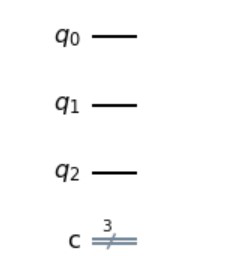This is how our circuit looks right now. We have three quantum bits and 3 classical bits, which will be used to measure the values of these Qubits, whenever we want. Right now they don’t have any value in them.

Step 2: Applying an X gate on the qubit which we have to teleport. We will also be adding a barrier, just to make the circuit more clear.

Now here, what we have done till now is that we have 3 qubits. What we’ll be doing is that we will be using q1 to transport data from q0 to q3. For this, we will use an X gate to init q0 to 1 state

## Python

 `circuit.x(``0``)  ``# used to apply an X gate.` `# This is done to make the circuit look more``# organized and clear.``circuit.barrier()``circuit.draw(output``=``'mpl'``)`

Output: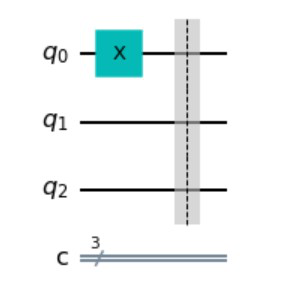This is how our circuit looks right now. On one of the Qubits, we have added an X gate, shown by the X. A barrier is added to make the circuit more organized as we keep on adding gates and other things. The classical bits are still untouched.

Step 3: Creating entanglement between Q1 and Q2 by applying a Hadamard gate on Q1, and a CX gate on Q1 and Q2 in such a way that the behavior of Q1 affects the behavior of Q2.

Here, what we’ll do is that we will create entanglement so that the behavior of the first qubit affects the behavior of the second qubit.

## Python

 `# This is how we apply a Hadamard gate on Q1.``circuit.h(``1``)` `# This is the CX gate, which takes two parameters,``# one being the control qubit and the``# other being the target qubit.``circuit.cx(``1``, ``2``)``circuit.draw(output``=``'mpl'``)`

Output: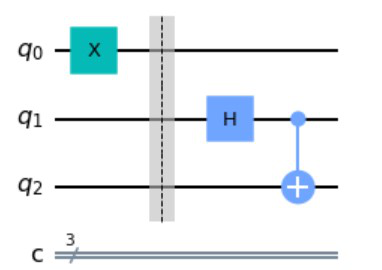Here we can see that after this step, this is how our circuit looks. We have a Hadamard gate applied to Q1, shown by the ‘H’ Symbol, and a Controlled NOT(CX) gate on Q1 and Q2. The classical bits are still untouched.

Creating entanglement between Q0 and Q1 by applying a Hadamard gate on Q0, and a CX gate on Q0 and Q1 in such a way that the behavior of Q1 affects the behavior of Q0. So essentially, we have a system where the behavior of either of the Qubits will affect the behavior of all the Qubits. Q1 can be considered as the portal we talked of, above. We will also now project the Qubits on the classical bits and measure the values of Q0 and Q1.

## Python

 `# The next step is to create a controlled``# gate between qubit 0 and qubit 1.``# Also we will be applying a Hadamard gate to q0.``circuit.cx(``0``, ``1``)``circuit.h(``0``)` `# Done for clarification of the circuit again.``circuit.barrier()` `# the next step is to do the two measurements``# on q0 and q1.``circuit.measure([``0``, ``1``], [``0``, ``1``])` `# circuit.measure can take any number of arguments,``# and has the following parameters:``# [qubit whose value is to be measured,``# classical bit where the value is stored]``circuit.draw(output``=``'mpl'``)`

Output: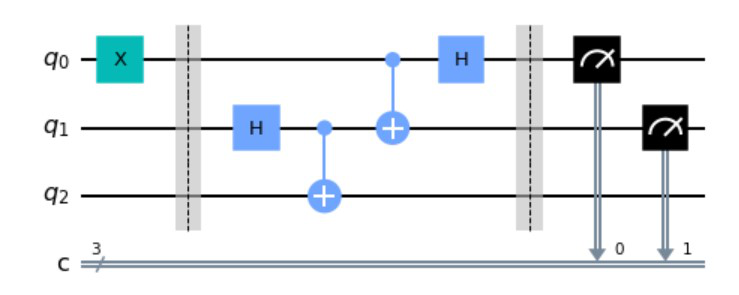Here we can see how our circuit looks. With the help of barriers, you can easily distinguish what was done in this step. We have a Hadamard gate applied to Q0, shown by the ‘H’ Symbol, and a Controlled NOT(CX) gate on Q0 and Q1. The classical bits are now put to use, with the black symbols showing that we have taken the value of Q0 and Q1 and stored it in classical bit 1 and classical bit 2.

The actual explanation will require an understanding of Quantum mechanics, so one can just understand that for a 00 measurement of the classical bits, we need to apply an I gate. For 01, an X gate needs to be applied, for 10, a Z gate needs to be applied and for 11, a ZX gate needs to be applied.  Since we don’t know what the value will be stored in classical bits, we are applying the more generalized Control X gates and Control Z gates.

The last step is to add two more gates, a controlled x gate and a controlled z gate (We have talked about this step in the text above, which tells what gates to apply for different measurements).

## Python

 `circuit.barrier()``circuit.cx(``1``, ``2``)``circuit.cz(``0``, ``2``)``circuit.draw(output``=``'mpl'``)`

Output: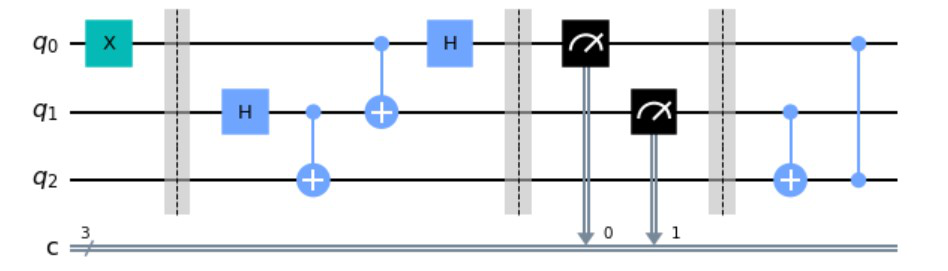This is the final required circuit for Quantum Teleportation. After the barrier, we can see that a Controlled X gate has been applied on Q1 and Q2, such that the behavior of Q2 affects the behavior of Q1. Also, a Control Z gate has been applied as shown between Q0 and Q2.

Now that our circuit has been made, all we need to do is to pass that circuit into a simulator so that we can get the results from the circuit back. Once we get the results back, we are plotting a histogram from the values of the classical bits that we have received. You can think of whatever we have done now as an Abstract Data Type created by us, and the code below is the main function where we will put it to use. Each step has been explained in complete detail for easy understanding of the reader.

## Python

 `# The first step is to call a simulator``# which we will use to perform simulations.``from` `qiskit.tools.visualization ``import` `plot_histogram``sim ``=` `Aer.get_backend(``'qasm_simulator'``)` `# here, like before, we have given the``# classical bit 2 the value of the Quantum bit 2.``circuit.measure(``2``, ``2``)` `# Now, we run the execute function,``# which takes our quantum circuit,``# the backend which we are using and``# the number of shots we want``# (shots are to increase accuracy and``# mitigate errors in Quantum Computing).``# All of this is stored in a variable called result` `result ``=` `execute(circuit, backend``=``sim, shots``=``1000``).result()``counts ``=` `result.get_counts()` `# This counts variable shows that for each possible combination,``# how many times the circuit gave a similar output``# (for example, 111 came x times, 101 came y times etc.)` `# importing plot_histogram which will help us visualize the results.``plot_histogram(counts)`

Output: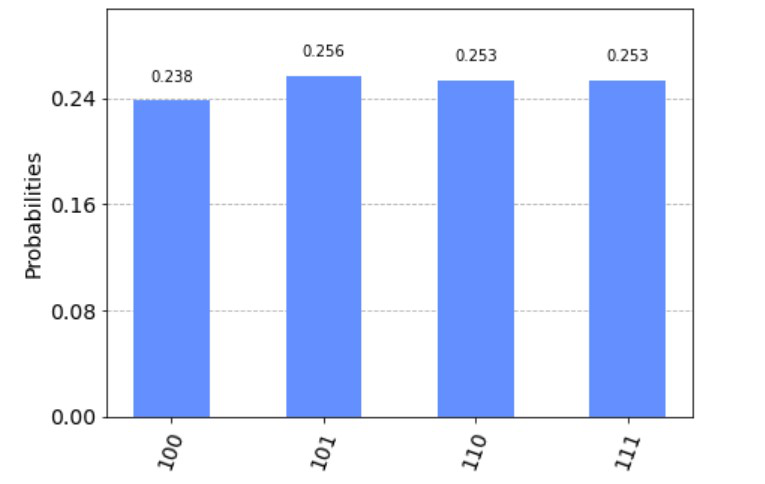## How does this prove that our algorithm worked?

Well, let’s see here. For 3 qubits, we can have states like 100, 101, 111, 001, and so on (8 of them to be precise, basic P&C stuff). Now, if I take one value from the x-axis, it is read in the following way:

 = [Value stored in classical bit 2, value stored in classical bit 1, value stored in classical bit 0]

We said that we will produce the same state on the first qubit on the second qubit. The state on the first qubit (the one which Sharanya had (Q0)) was 1, And look here, we have only received the permutations, where the value of the second classical bit is 1, which proves that the value on the second qubit (the one which Kartik had (Q2)) was 1 as well!

My Personal Notes arrow_drop_up